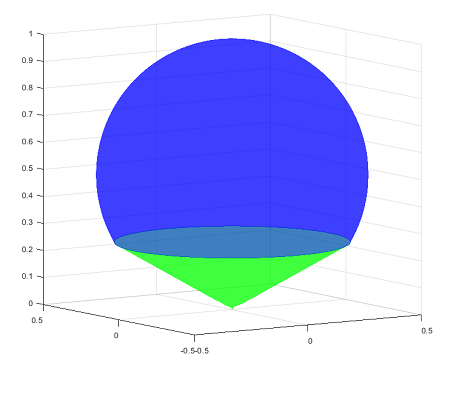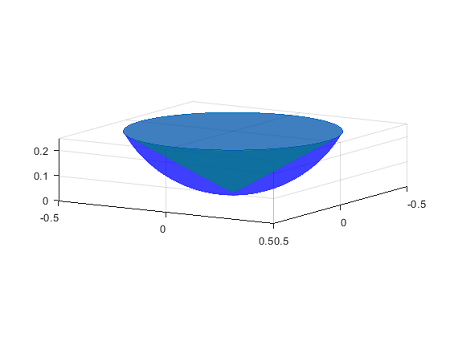# Consider the solid bounded by the sphere x^2 + y^2 + z^2 = z that is above the cone Z = (1 /...

## Question:

Consider the solid bounded by the sphere {eq}x^2 + y^2 + z^2 = z {/eq} that is above the cone {eq}Z = (\frac{1}{\sqrt {3}}) \sqrt {x^2 + y^2} {/eq}.

Sketch the solid and use cylindrical coordinates to find the volume of the solid.

## Volume of the solid bounded between two surfaces

The volume of the region bounded between two surfaces is given by integrating the absolute value of the difference of the two functions determining the two surfaces over the region. Usually, the given surfaces are in form of functions (or an expression of a variable in terms of the two other variables). For surfaces with some radial symmetry, one can exploit this symmetry to make the computation easier by re-writing the integral in cylindrical coordinates or spherical coordinates.

Let {eq}(S) {/eq} and {eq}(C) {/eq} be the sphere and the cone respectively. In cylindrical coordinate, we have {eq}x=r\sin \theta, y=r\cos\theta \quad (r\ge 0, \theta\in[0,2\pi)) {/eq}

First, for the sphere

{eq}x^2+y^2+z^2=z\implies x^2+y^2+z^2-z=0 \implies x^2+y^2+\left(z^2-z+\frac14\right)=\frac 14 \implies x^2+y^2+\left(z-\frac12\right)^2=\left(\frac12\right)^2 {/eq}

so the sphere is centered at {eq}(0,0,1/2) {/eq} and the radius is {eq}1/2 {/eq}. Noting that {eq}x^2+y^2=r^2 {/eq}, so in the cylindrical coordinates, the equation of the sphere is

Similarly, for the cone, we have

Here, there is no restriction the angle of rotation, so {eq}0\le \theta< 2\pi {/eq},

The intersection of the two given surfaces is determined by

{eq}\begin{cases} &r^2+z^2=z\\ &r=z\sqrt 3 \end{cases} \implies \begin{cases} &r^2=z-z^2\\ &r^2= 3z^2 \end{cases} \implies \begin{cases} &3z^2=z-z^2\\ &r=z\sqrt 3 \end{cases}\\ \implies \begin{cases} &4z^2-z=0\\ &r^2=3z^2 \end{cases} \implies \begin{cases} &z(4z-1)=0\\ &r^2=3z^2 \end{cases} \implies \begin{cases} &z=0 \quad\text{or} \quad z=\frac14 \\ &r=z\sqrt{3} \end{cases}\\ \implies \begin{cases} &z=0\\ &r=0 \end{cases}\quad\text{or} \quad \begin{cases} &z=\frac14 \\ &r^2=x^2+y^2=\frac 3{16} \end{cases}\\ {/eq}

(That is, the origin, and the circle given by {eq}(x^2+y^2=3/16,\quad z=1/4) {/eq})

The following is the picture of the solid {eq}D {/eq} bounded between the sphere and the cone that is above the coneOne strategy to compute the volume of {eq}D {/eq} is to subtract the volume of the solid {eq}D' {/eq} bounded between the cone and the sphere which lies below the cone from the volume of the sphere, where for the first quantity, we can use technique in calculus.

Let {eq}f(z,\theta)=3z^2 {/eq} and {eq}g(z,\theta)=z-z^2 {/eq}. The equations of the cone and the sphere are then {eq}f(z,\theta)=r^2 {/eq} and {eq}g(z,\theta)=r^2 {/eq} respectively.

The following is the picture of the solid D' bounded between the sphere and the cone that is below the coneThe volume form of The volume of the solid {eq}D' {/eq} is given by

The volume of the solid {eq}D' {/eq} bounded between the two surfaces is

{eq}\int_D dV\\ =\frac12\int_0^{2\pi}\int_{0}^{1/4}(z-4z^2)dz d\theta\\ =\frac12\int_0^{2 \pi}d\theta\int_{0}^{1/4}\int_{z\sqrt 3}^{\sqrt{z-z^2}}(z-4z^2)dz\\ =\pi\int_{0}^{1/4}(z-4z^2)dz\\ =\pi(\frac{z^2}2-\frac{4z^3}3)|_0^{1/4} =\pi\times(\frac1{16}-\frac{4}3\times \frac1{64})\\ =\frac\pi{96} {/eq}

Hence, the volume of the solid {eq}D {/eq} we need to compute is:

{eq}\frac43\pi(\text{radius })^3-\text{(Volume of } D'\text{)}\\ =\frac43\pi(\frac12)^3-\frac{\pi}{96}\\ =\frac{5}{32}\pi {/eq}